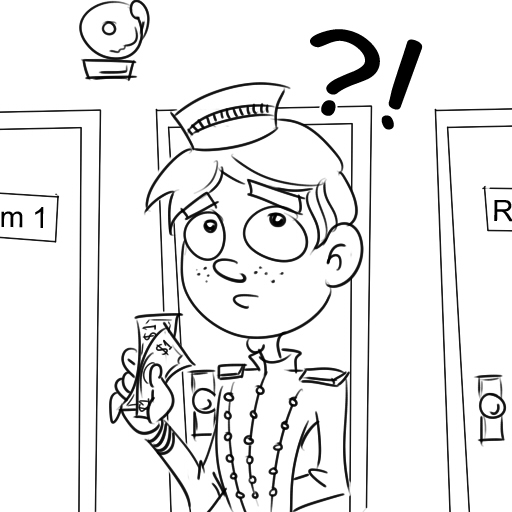# The Missing Dollar!

Algebra Level 1Three men go to stay at a motel, and the man at the desk charges them $30.00 for a room. They split the cost ten dollars each. Later the manager tells the desk man that he overcharged the men, that the actual cost should have been$25.00. The manager gives the bellboy $5.00 and tells him to give it to the men. The bellboy, however, decides to cheat the men and pockets$2.00, giving each of the men only one dollar.

Now each man has paid $9.00 to stay in the room and 3 x$9.00 = $27.00. The bellboy has pocketed$2.00. $27.00 +$2.00 = $29.00 - so where is the missing$1.00?

×